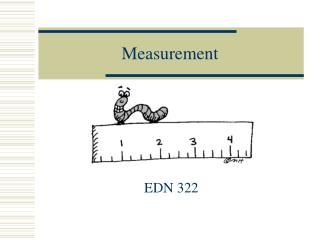Download PresentationMeasurementMeasurement - PowerPoint PPT Presentation

Download PresentationMeasurement
An Image/Link below is provided (as is) to download presentation

Download Policy: Content on the Website is provided to you AS IS for your information and personal use and may not be sold / licensed / shared on other websites without getting consent from its author. While downloading, if for some reason you are not able to download a presentation, the publisher may have deleted the file from their server.

- - - - - - - - - - - - - - - - - - - - - - - - - - - E N D - - - - - - - - - - - - - - - - - - - - - - - - - - -
Presentation Transcript

1. Measurement EDN 322

2. Why teach measurement? • Provides many applications to everyday life • Can be used to help learn other topics in mathematics • Can be related to other areas of the school curriculum • Involves students in active learning • Can be approached through problem solving

3. Teaching Measurement • Children must measure frequently and often. • Children must develop estimation skills with measurement. • Children should encounter activity-oriented measurement situations by doing and experimenting rather than by passively observing.

4. Measurement Attributes • Length • Perimeter • Area • Area • Capacity • Volume • Weight • Time • Temperature • Money

5. Stages for teaching measurement • Identify the attribute by comparing and ordering objects • Perceptually • Directly • Indirectly • Choose a unit • Nonstandard units • Standard units of measurement • Compare the object to the unit • Find the number of units • Report the number of units

6. Concepts Related to Units • A measurement must include both a number and the unit • Two measurements may be easily compared if the same unit is used. • One unit may be more appropriate than another to measure an object.

7. Concepts Related to Units • There is an inverse relationship between the number of units and the size of the unit. • A smaller unit will give a more exact measurement. • Standard units are needed to communicate effectively.

8. NCTM • Understand measurable attributes of objects and the units, systems, and processes of measurement • Apply appropriate techniques, tools, and formulas to determine measurements

9. NC SCOS Kindergarten 2.01 Compare attributes of two objects using appropriate vocabulary (color, weight, height, width, length, texture). 2.02 Recognize concepts of calendar time using appropriate vocabulary (days of the week, months of the year, seasons).

10. NC SCOSFirst Grade • 2.01 For given objects: a) Select an attribute (length, capacity, mass) to measure (use non-standard units). b) Develop strategies to estimate size. c) Compare, using appropriate language, with respect to the attribute selected. • 2.02 Develop an understanding of the concept of time. a) Tell time at the hour and half-hour. b) Solve problems involving applications of time (clock and calendar).

11. NC SCOSSecond Grade • 2.01 Estimate and measure using appropriate units. a) Length (meters, centimeters, feet, inches, yards). b) Temperature (Fahrenheit). • 2.02 Tell time at the five-minute intervals.

12. NC SCOSThird Grade • 2.01 Solve problems using measurement concepts and procedures involving: a) Elapsed time. b) Equivalent measures within the same measurement system. • 2.02 Estimate and measure using appropriate units. a) Capacity (cups, pints, quarts, gallons, liters). b) Length (miles, kilometers) c) Mass (ounces, pounds, grams, kilograms). d) Temperature (Fahrenheit, Celsius).

13. NC SCOSFourth Grade • 2.01 Develop strategies to determine the area of rectangles and the perimeter of plane figures. • 2.02 Solve problems involving perimeter of plane figures and areas of rectangles.

14. NC SCOSFifth Grade • 2.01 Estimate the measure of an object in one system given the measure of that object in another system. • 2.02 Identify, estimate, and measure the angles of plane figures using appropriate tools.